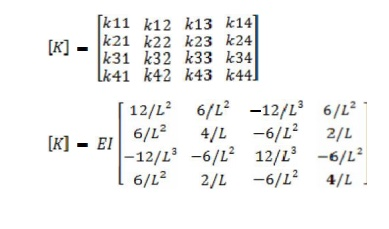Home | | Structural Analysis I | Important Questions and Answers: Structural Analysis - Stiffness Matrix Method

# Important Questions and Answers: Structural Analysis - Stiffness Matrix Method

Civil - Structural Analysis - Stiffness Matrix Method

STIFFNESS MATRIX METHOD

1. What are the basic unknowns in stiffness matrix method?

In the stiffness matrix method nodal displacements are treated as the basic unknowns for the solution of indeterminate structures.

2. Define stiffness coefficient kij.

Stiffness coefficient 'kij' is defined as the force developed at joint 'i' due to unit displacement at joint 'j' while all other joints are fixed.

3. What is the basic aim of the stiffness method?

The aim of the stiffness method is to evaluate the values of generalized coor dinates 'r' knowing the structure stiffness matrix 'k' and nodal loads 'R' through the structure equilibrium equation.

{R} =[K]{r}

4. What is the displacement transformation matrix?

The connectivity matrix which relates the internal displacement 'q' and the external displacement 'r' is known as the displacement transformation matrix 'a'.

{q} =[a]{r}

5. How are the basic equations of stiffness matrix obtained?

The basic equations of stiffness matrix are obtained as:

Equilibrium forces

�Compatibility of displacements

Force displacement relationships

6. What is the equilibrium condition used in the stiffness method?

The external loads and the internal member forces must be in equilibrium at the nodal points.

7. What is meant by generalized coordinates?

For specifying a configuration of a system, a certain minimum no of indepen dent coordinates are necessary. The least no of independent coordinates that are needed to specify the configuration is known as generalized coordinates.

8. What is the compatibility condition used in the flexibility method?

The deformed elements fit together at nodal points.

9. Write about the force displacement relationship.

The relationship of each element must satisfy the stress-strain relationship of the element material.

10.            Write the element stiffness for a truss element.

The element stiffness matrix for a truss element is given by11.            Write the element stiffness matrix for a beam element.

The element stiffness matrix for a beam element is given by12.            Compare flexibility method and stiffness method.

Flexibility matrix method

The redundant forces are treated as basic unknowns.

The number of equations involved is equal to the degree of static indete rminacy of the structure.

The method is the generalization of consistent deformation method. Different procedures are used for determinate and indeterminate structures

Stiffness matrix method

The joint displacements are treated as basic unknowns

The number of displacements involved is equal to the no of degrees of freedom of the structure

The method is the generalization of the slope deflection method.

The same procedure is used for both determinate and indeterminate structures.

13.            Is it possible to develop the flexibility matrix for an unstable structure?

In order to develop the flexibility matrix for a structure, it has to be stable and determinate.

14.            What is the relation between flexibility and stiffness matrix?

The element stiffness matrix 'k' is the inverse of the element flexibility matrix 'f' and is given by f=1/k or k =1/f.

15.            What are the type of structtures that can be solved using stiffness matrix method?

Structures such as simply supported, fixed beams and portal frames can be solved using stiffness matrix method.

16.            Give the formula for the size of the Global stiffness matrix.

The size of the global stiffness matrix (GSM) =No: of nodes x Degrees of free dom per node.

17.            List the properties of the stiffness matrix

The properties of the stiffness matrix are:

It is a symmetric matrix

The sum of elements in any column must be equal to zero.

It is an unstable element there fore the determinant is equal to zero.

18Why is the stiffness matrix method also called equilibrium method or displacement method?

Stiffness method is based on the superposition of displacements and hence is also known as the dispalcement method. And since it leads to the equilibrium equations the method is also known as equilibrium method.

19 If the flexibility matrix is given as20 Write the n stiffness matrix for a 2D beam element. The stiffness matrix for a 2 D beam element is given byStudy Material, Lecturing Notes, Assignment, Reference, Wiki description explanation, brief detail
Civil : Structural Analysis : Stiffness Matrix Method : Important Questions and Answers: Structural Analysis - Stiffness Matrix Method |